Скачать презентацию 18739 A Foundations of Security and Privacy Logics

97f5f534d0a4f0281f0e420f29f6d915.ppt

• Количество слайдов: 33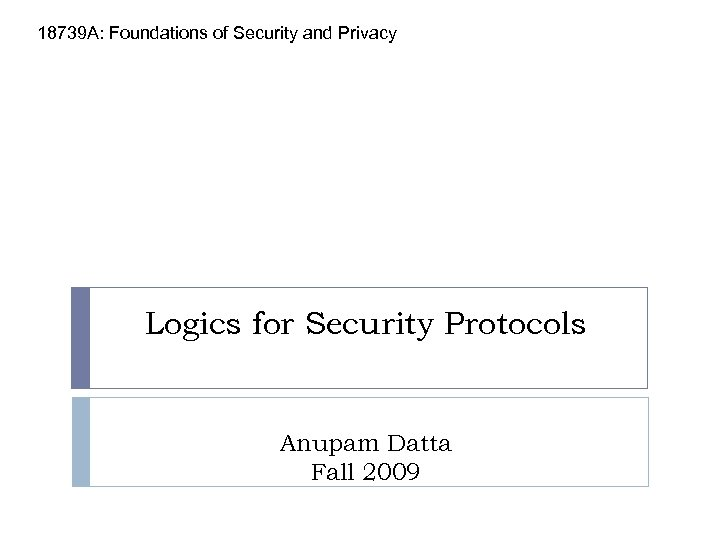18739 A: Foundations of Security and Privacy Logics for Security Protocols Anupam Datta Fall 2009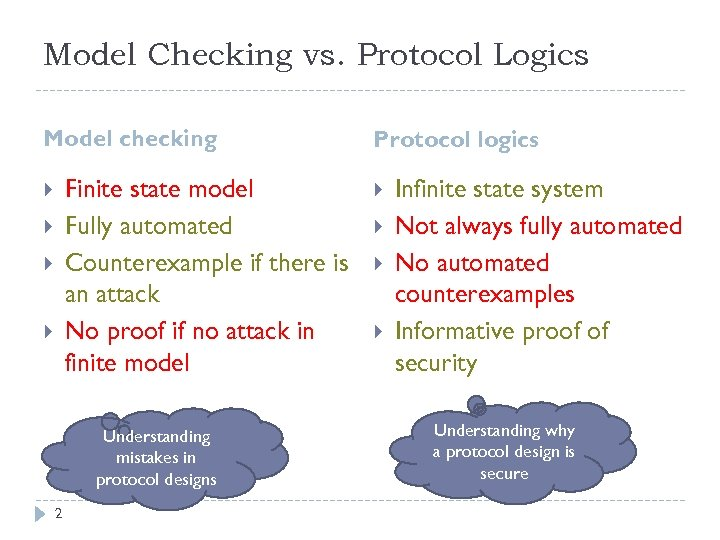Model Checking vs. Protocol Logics Model checking Finite state model Fully automated Counterexample if there is an attack No proof if no attack in finite model Understanding mistakes in protocol designs 2 Protocol logics Infinite state system Not always fully automated No automated counterexamples Informative proof of security Understanding why a protocol design is secure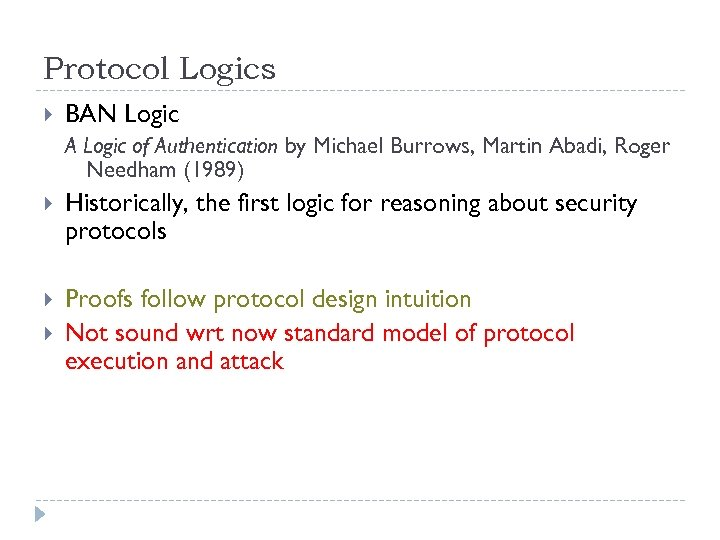Protocol Logics BAN Logic A Logic of Authentication by Michael Burrows, Martin Abadi, Roger Needham (1989) Historically, the first logic for reasoning about security protocols Proofs follow protocol design intuition Not sound wrt now standard model of protocol execution and attack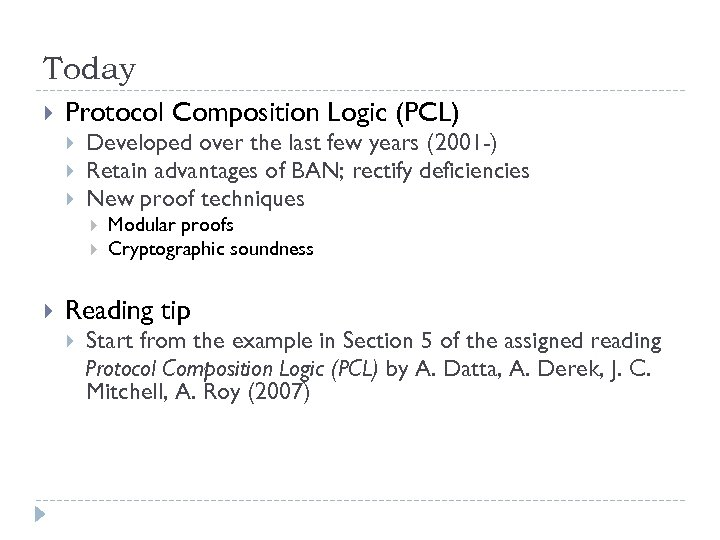Today Protocol Composition Logic (PCL) Developed over the last few years (2001 -) Retain advantages of BAN; rectify deficiencies New proof techniques Modular proofs Cryptographic soundness Reading tip Start from the example in Section 5 of the assigned reading Protocol Composition Logic (PCL) by A. Datta, A. Derek, J. C. Mitchell, A. Roy (2007)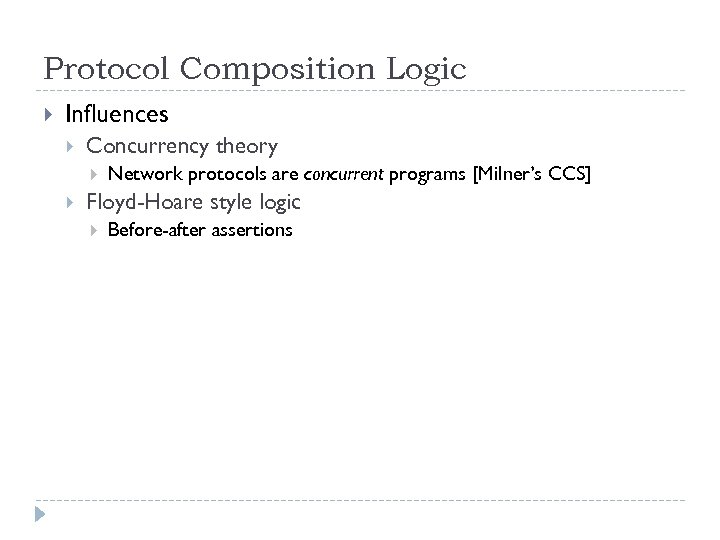Protocol Composition Logic Influences Concurrency theory Network protocols are concurrent programs [Milner’s CCS] Floyd-Hoare style logic Before-after assertions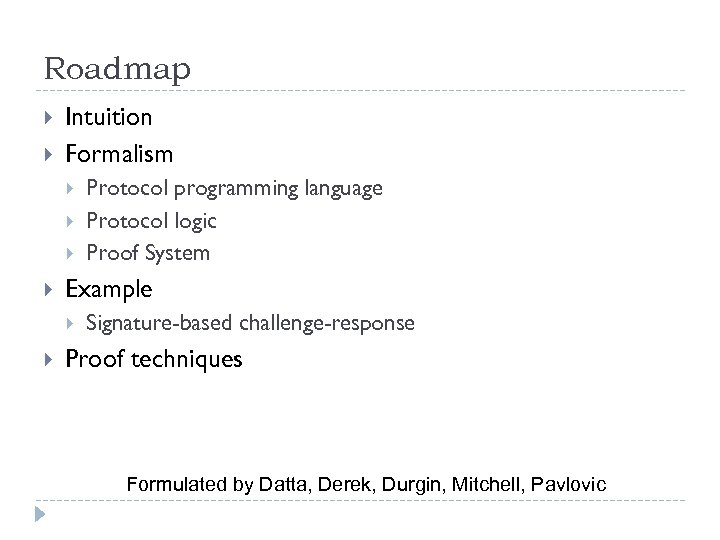Roadmap Intuition Formalism Example Protocol programming language Protocol logic Proof System Signature-based challenge-response Proof techniques Formulated by Datta, Derek, Durgin, Mitchell, Pavlovic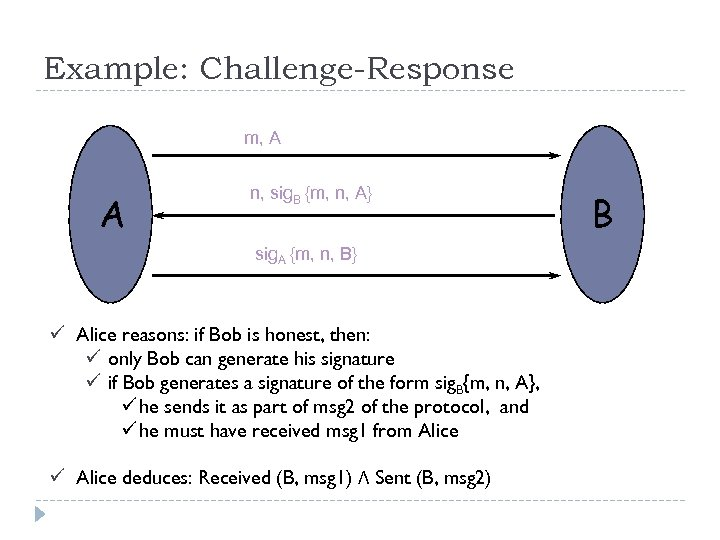Example: Challenge-Response m, A A n, sig. B {m, n, A} sig. A {m, n, B} ü Alice reasons: if Bob is honest, then: ü only Bob can generate his signature ü if Bob generates a signature of the form sig. B{m, n, A}, ü he sends it as part of msg 2 of the protocol, and ü he must have received msg 1 from Alice ü Alice deduces: Received (B, msg 1) Λ Sent (B, msg 2) B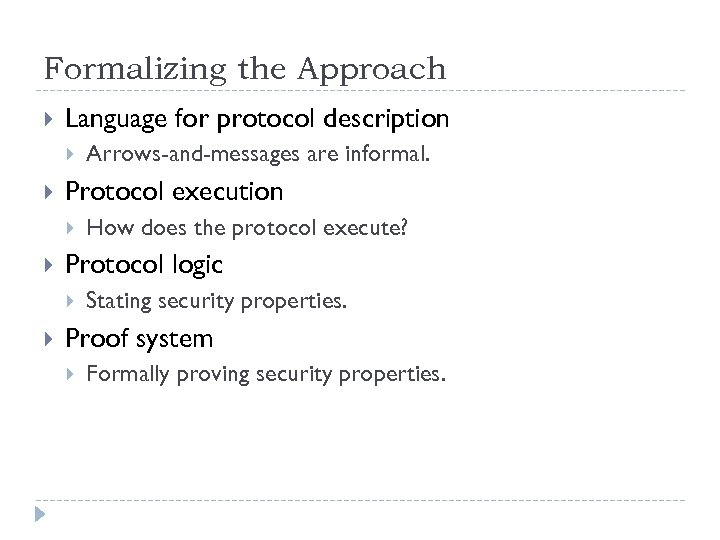Formalizing the Approach Language for protocol description Protocol execution How does the protocol execute? Protocol logic Arrows-and-messages are informal. Stating security properties. Proof system Formally proving security properties.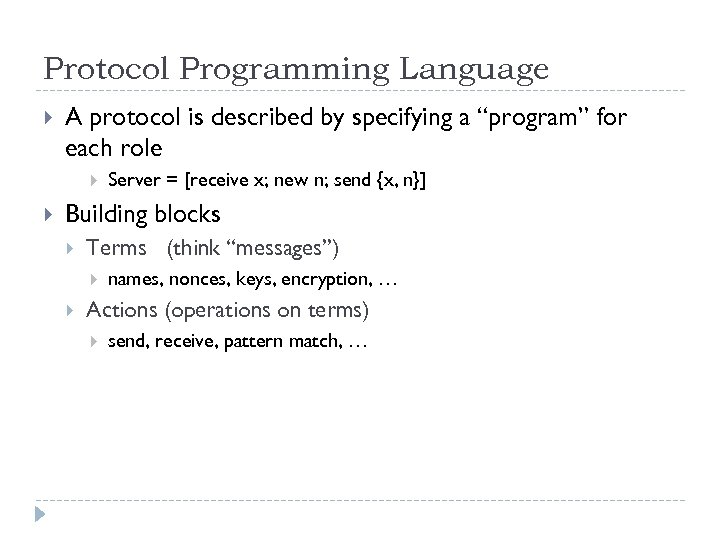Protocol Programming Language A protocol is described by specifying a “program” for each role Server = [receive x; new n; send {x, n}] Building blocks Terms (think “messages”) names, nonces, keys, encryption, … Actions (operations on terms) send, receive, pattern match, …Terms t : : = c x N K t, t sig. K{t} enc. K{t} constant term variable name key tupling signature encryption Example: x, sig. B{m, x, A} is a term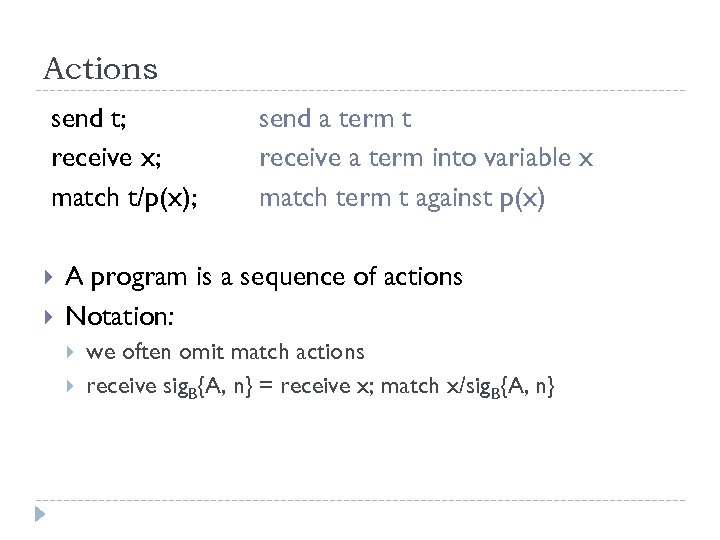Actions send t; receive x; match t/p(x); send a term t receive a term into variable x match term t against p(x) A program is a sequence of actions Notation: we often omit match actions receive sig. B{A, n} = receive x; match x/sig. B{A, n}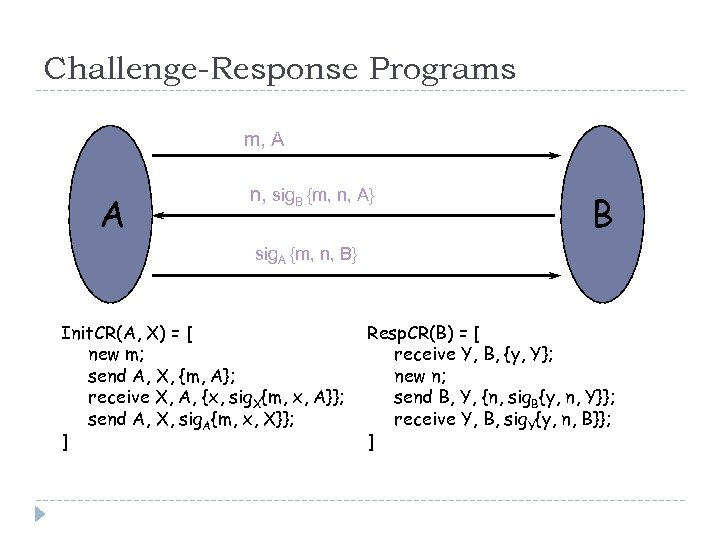Challenge-Response Programs m, A A n, sig. B {m, n, A} B sig. A {m, n, B} Init. CR(A, X) = [ new m; send A, X, {m, A}; receive X, A, {x, sig. X{m, x, A}}; send A, X, sig. A{m, x, X}}; ] Resp. CR(B) = [ receive Y, B, {y, Y}; new n; send B, Y, {n, sig. B{y, n, Y}}; receive Y, B, sig. Y{y, n, B}}; ]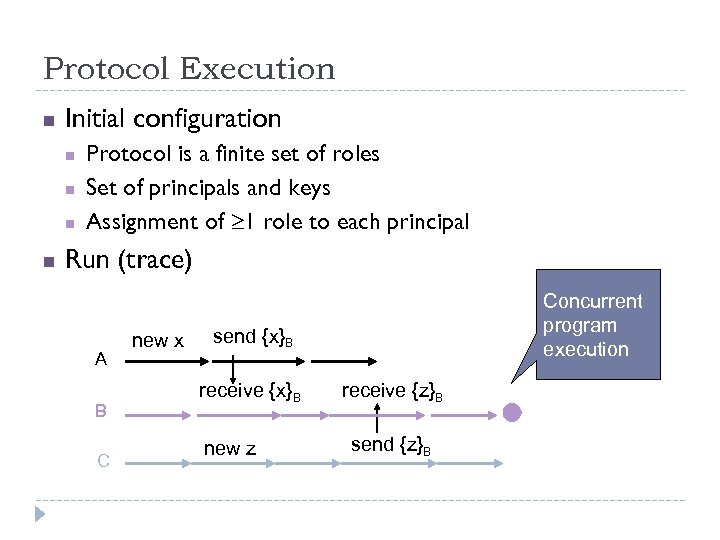Protocol Execution n Initial configuration n n Protocol is a finite set of roles Set of principals and keys Assignment of 1 role to each principal Run (trace) A B C new x Concurrent program execution send {x}B receive {x}B new z receive {z}B send {z}B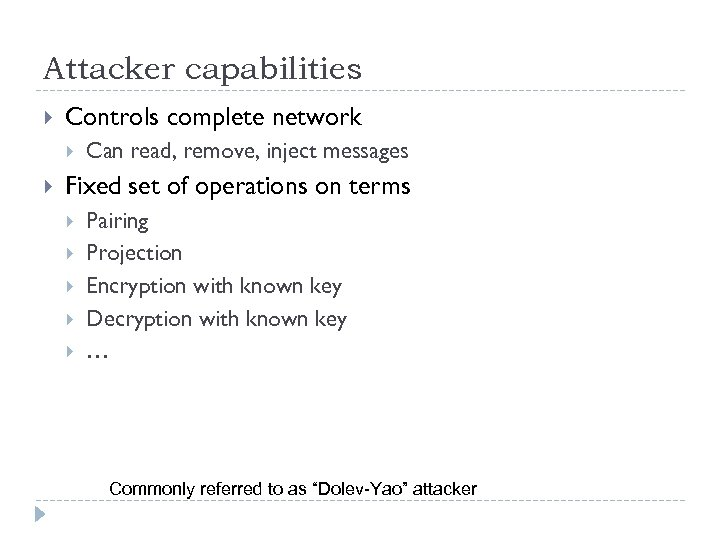Attacker capabilities Controls complete network Can read, remove, inject messages Fixed set of operations on terms Pairing Projection Encryption with known key Decryption with known key … Commonly referred to as “Dolev-Yao” attacker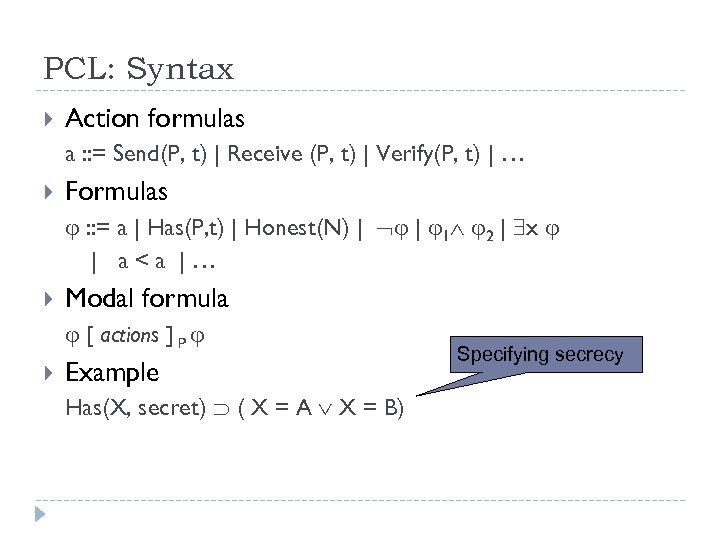PCL: Syntax Action formulas a : : = Send(P, t) | Receive (P, t) | Verify(P, t) | … Formulas : : = a | Has(P, t) | Honest(N) | | 1 2 | x | a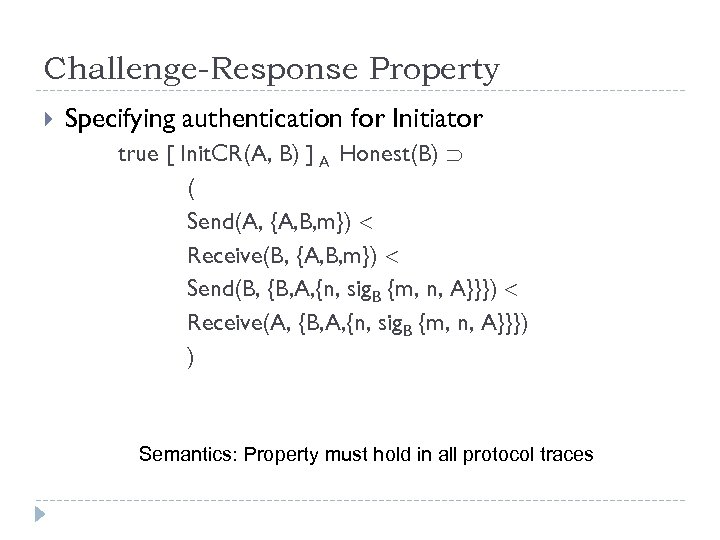Challenge-Response Property Specifying authentication for Initiator true [ Init. CR(A, B) ] A Honest(B) ( Send(A, {A, B, m}) Receive(B, {A, B, m}) Send(B, {B, A, {n, sig. B {m, n, A}}}) Receive(A, {B, A, {n, sig. B {m, n, A}}}) ) Semantics: Property must hold in all protocol traces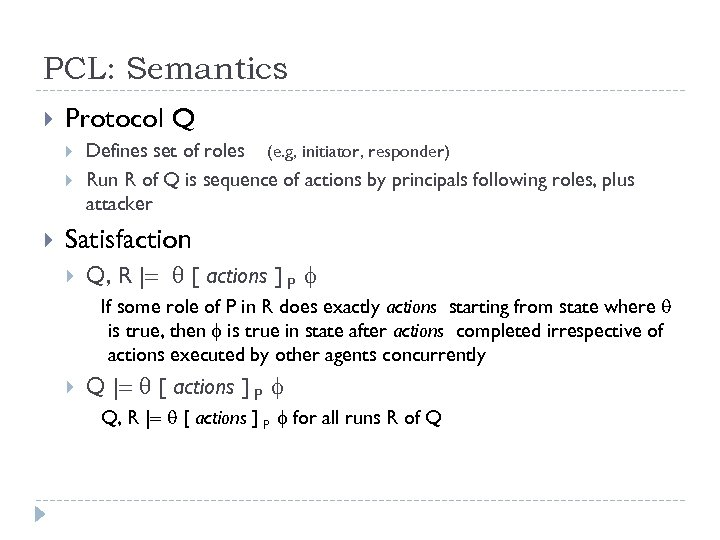PCL: Semantics Protocol Q Defines set of roles (e. g, initiator, responder) Run R of Q is sequence of actions by principals following roles, plus attacker Satisfaction Q, R | [ actions ] P If some role of P in R does exactly actions starting from state where is true, then is true in state after actions completed irrespective of actions executed by other agents concurrently Q | [ actions ] P Q, R | [ actions ] P for all runs R of Q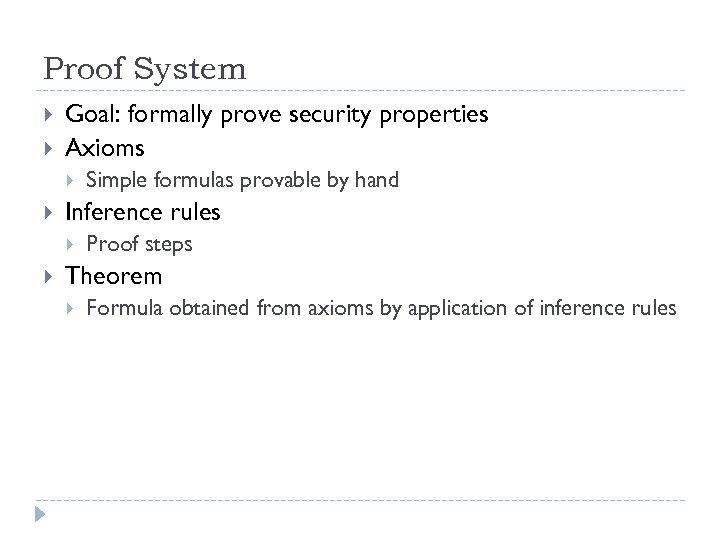Proof System Goal: formally prove security properties Axioms Inference rules Simple formulas provable by hand Proof steps Theorem Formula obtained from axioms by application of inference rules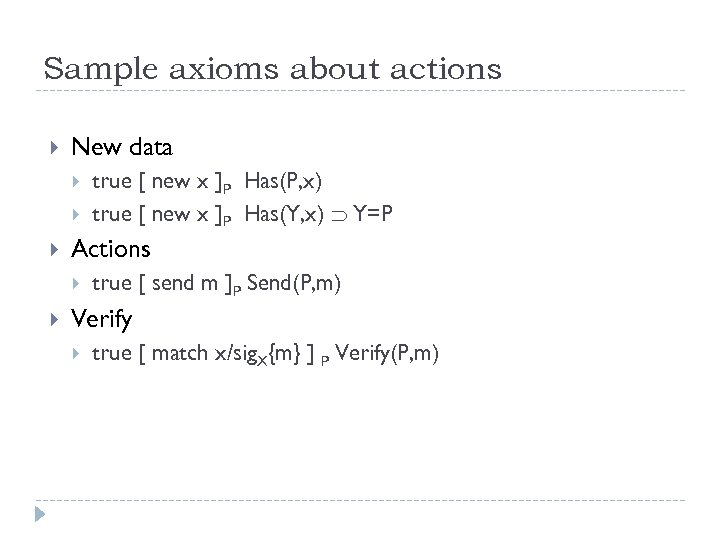Sample axioms about actions New data Actions true [ new x ]P Has(P, x) true [ new x ]P Has(Y, x) Y=P true [ send m ]P Send(P, m) Verify true [ match x/sig. X{m} ] P Verify(P, m)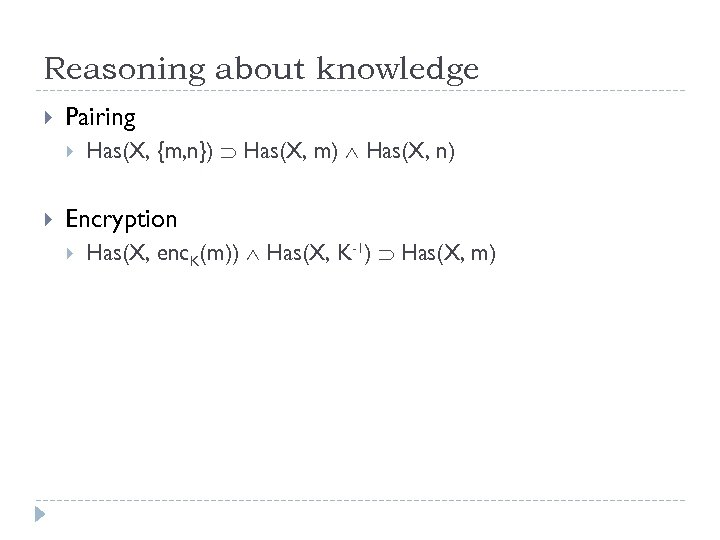Reasoning about knowledge Pairing Has(X, {m, n}) Has(X, m) Has(X, n) Encryption Has(X, enc. K(m)) Has(X, K-1) Has(X, m)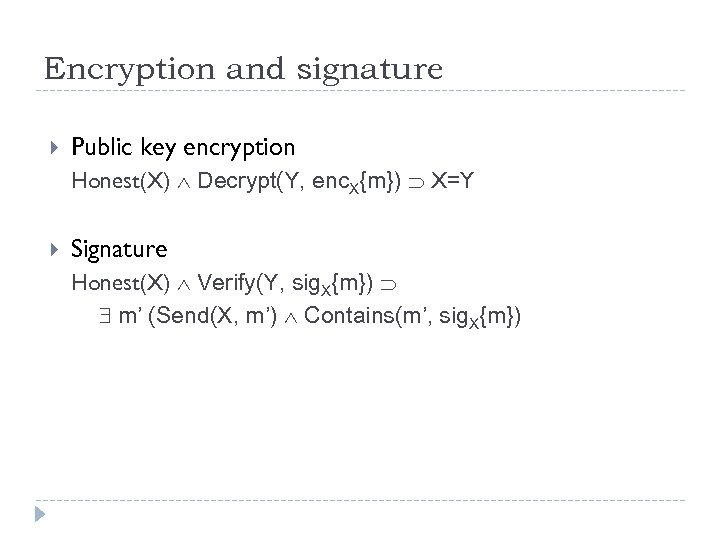Encryption and signature Public key encryption Honest(X) Decrypt(Y, enc. X{m}) X=Y Signature Honest(X) Verify(Y, sig. X{m}) m’ (Send(X, m’) Contains(m’, sig. X{m})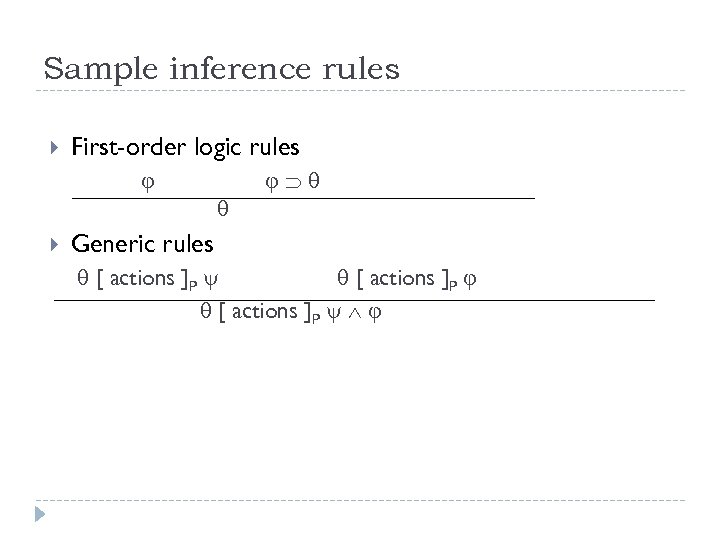Sample inference rules First-order logic rules Generic rules [ actions ]P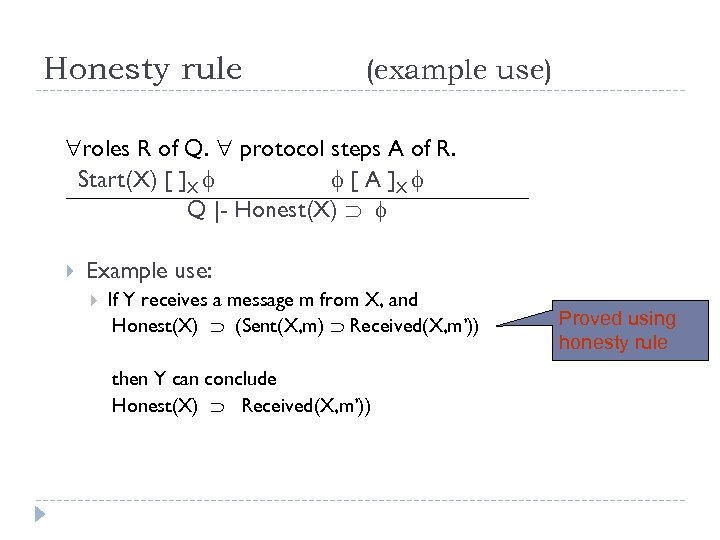Honesty rule (example use) roles R of Q. protocol steps A of R. Start(X) [ ]X [ A ]X Q |- Honest(X) Example use: If Y receives a message m from X, and Honest(X) (Sent(X, m) Received(X, m’)) then Y can conclude Honest(X) Received(X, m’)) Proved using honesty rule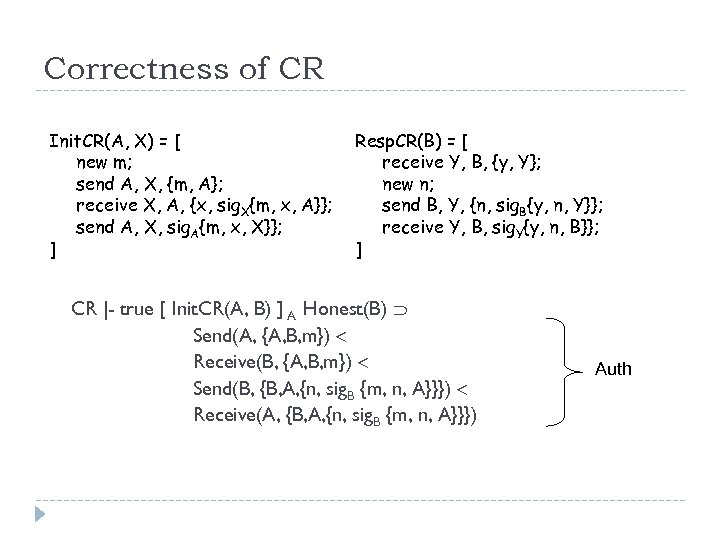Correctness of CR Init. CR(A, X) = [ new m; send A, X, {m, A}; receive X, A, {x, sig. X{m, x, A}}; send A, X, sig. A{m, x, X}}; ] Resp. CR(B) = [ receive Y, B, {y, Y}; new n; send B, Y, {n, sig. B{y, n, Y}}; receive Y, B, sig. Y{y, n, B}}; ] CR |- true [ Init. CR(A, B) ] A Honest(B) Send(A, {A, B, m}) Receive(B, {A, B, m}) Send(B, {B, A, {n, sig. B {m, n, A}}}) Receive(A, {B, A, {n, sig. B {m, n, A}}}) Auth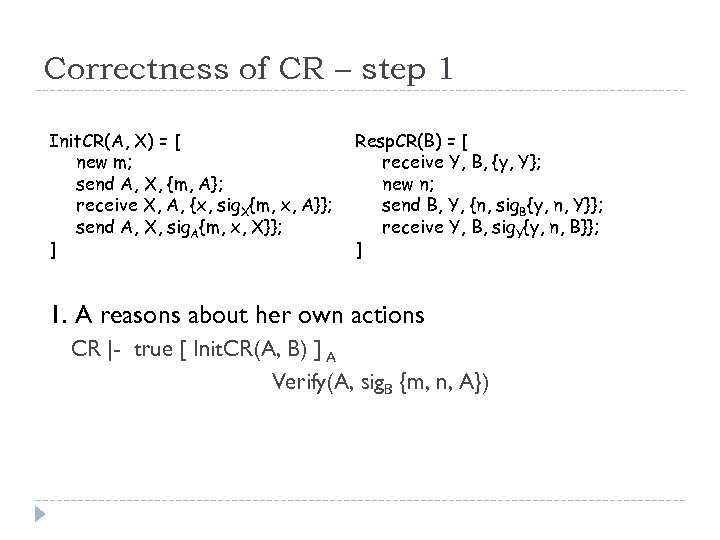Correctness of CR – step 1 Init. CR(A, X) = [ new m; send A, X, {m, A}; receive X, A, {x, sig. X{m, x, A}}; send A, X, sig. A{m, x, X}}; ] Resp. CR(B) = [ receive Y, B, {y, Y}; new n; send B, Y, {n, sig. B{y, n, Y}}; receive Y, B, sig. Y{y, n, B}}; ] 1. A reasons about her own actions CR |- true [ Init. CR(A, B) ] A Verify(A, sig. B {m, n, A})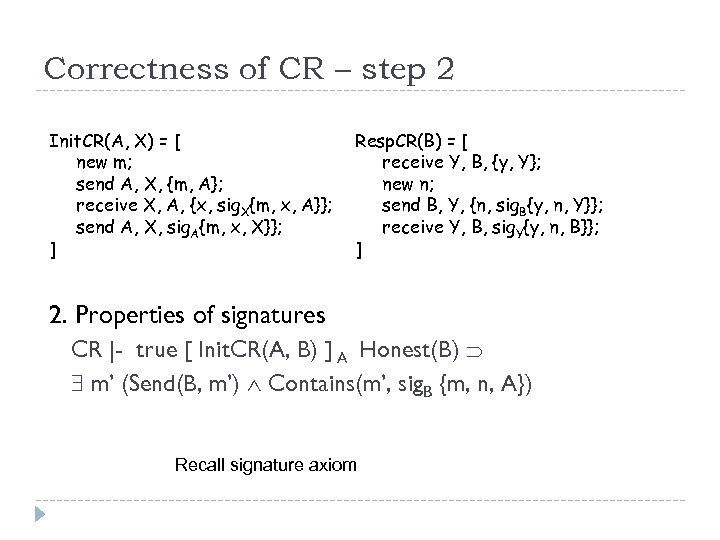Correctness of CR – step 2 Init. CR(A, X) = [ new m; send A, X, {m, A}; receive X, A, {x, sig. X{m, x, A}}; send A, X, sig. A{m, x, X}}; ] Resp. CR(B) = [ receive Y, B, {y, Y}; new n; send B, Y, {n, sig. B{y, n, Y}}; receive Y, B, sig. Y{y, n, B}}; ] 2. Properties of signatures CR |- true [ Init. CR(A, B) ] A Honest(B) m’ (Send(B, m’) Contains(m’, sig. B {m, n, A}) Recall signature axiom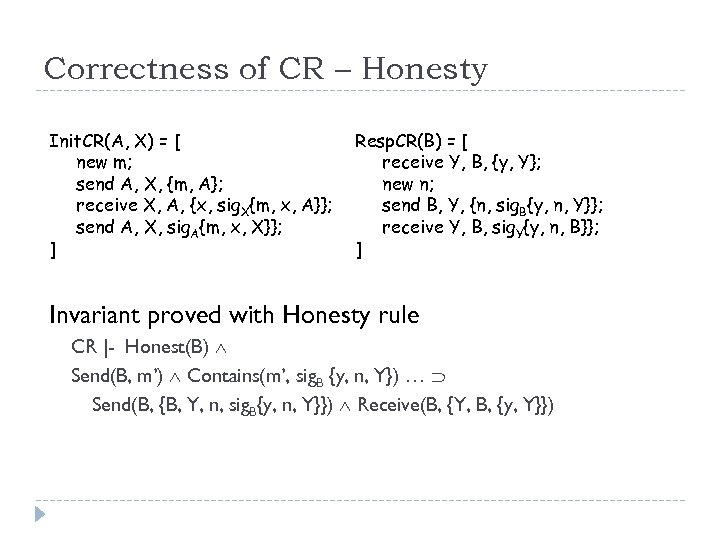Correctness of CR – Honesty Init. CR(A, X) = [ new m; send A, X, {m, A}; receive X, A, {x, sig. X{m, x, A}}; send A, X, sig. A{m, x, X}}; ] Resp. CR(B) = [ receive Y, B, {y, Y}; new n; send B, Y, {n, sig. B{y, n, Y}}; receive Y, B, sig. Y{y, n, B}}; ] Invariant proved with Honesty rule CR |- Honest(B) Send(B, m’) Contains(m’, sig. B {y, n, Y}) … Send(B, {B, Y, n, sig. B{y, n, Y}}) Receive(B, {Y, B, {y, Y}})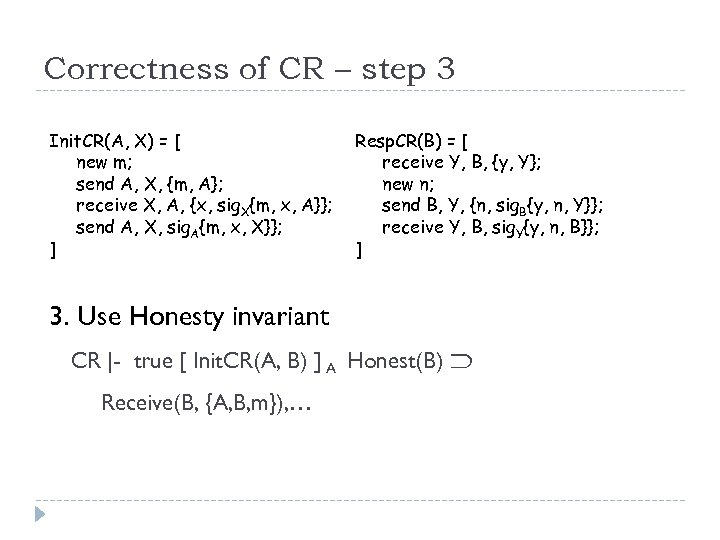Correctness of CR – step 3 Init. CR(A, X) = [ new m; send A, X, {m, A}; receive X, A, {x, sig. X{m, x, A}}; send A, X, sig. A{m, x, X}}; ] Resp. CR(B) = [ receive Y, B, {y, Y}; new n; send B, Y, {n, sig. B{y, n, Y}}; receive Y, B, sig. Y{y, n, B}}; ] 3. Use Honesty invariant CR |- true [ Init. CR(A, B) ] A Honest(B) Receive(B, {A, B, m}), …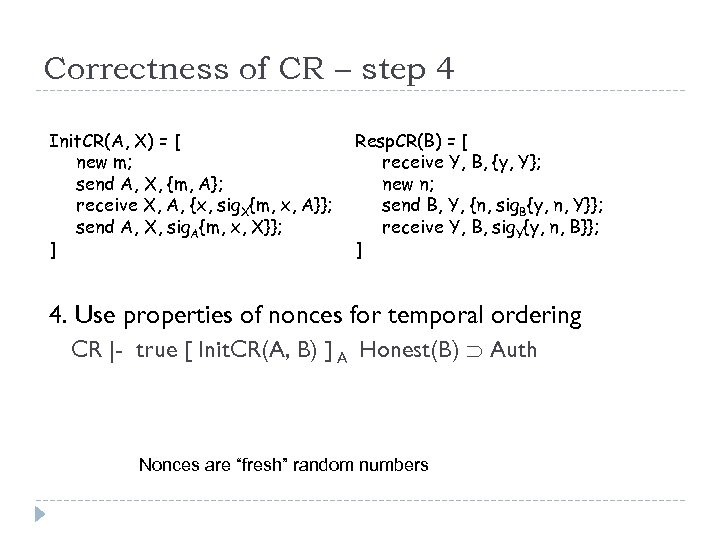Correctness of CR – step 4 Init. CR(A, X) = [ new m; send A, X, {m, A}; receive X, A, {x, sig. X{m, x, A}}; send A, X, sig. A{m, x, X}}; ] Resp. CR(B) = [ receive Y, B, {y, Y}; new n; send B, Y, {n, sig. B{y, n, Y}}; receive Y, B, sig. Y{y, n, B}}; ] 4. Use properties of nonces for temporal ordering CR |- true [ Init. CR(A, B) ] A Honest(B) Auth Nonces are “fresh” random numbers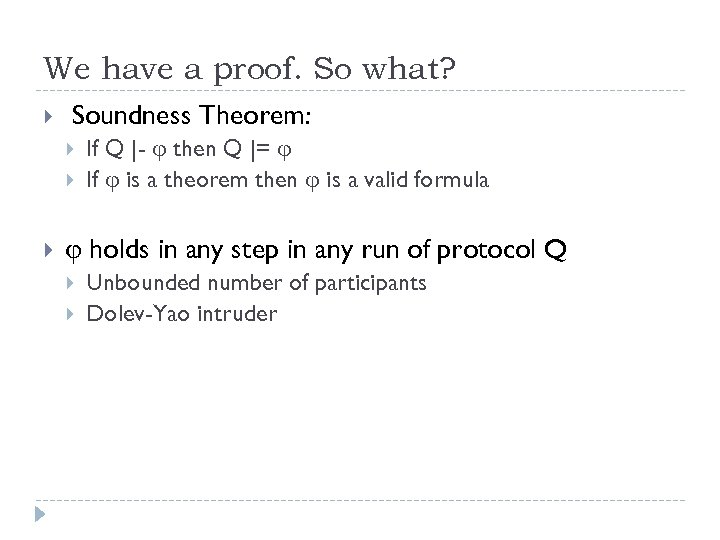We have a proof. So what? Soundness Theorem: If Q |- then Q |= If is a theorem then is a valid formula holds in any step in any run of protocol Q Unbounded number of participants Dolev-Yao intruderThanks ! Questions?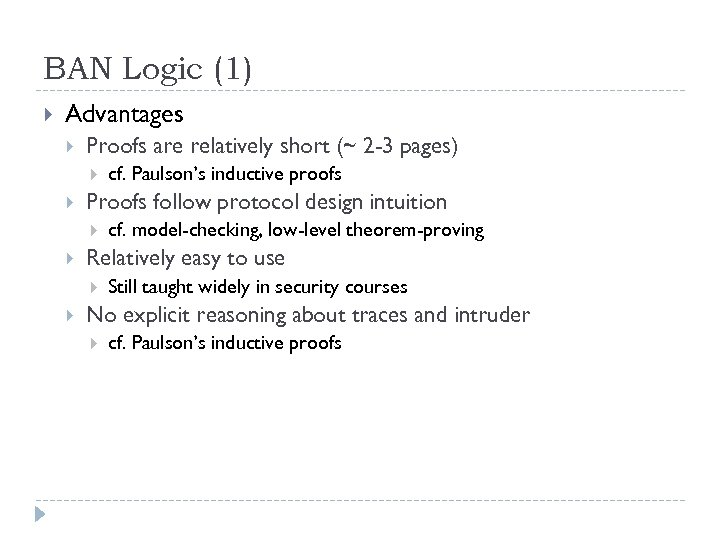BAN Logic (1) Advantages Proofs are relatively short (~ 2 -3 pages) Proofs follow protocol design intuition cf. model-checking, low-level theorem-proving Relatively easy to use cf. Paulson’s inductive proofs Still taught widely in security courses No explicit reasoning about traces and intruder cf. Paulson’s inductive proofs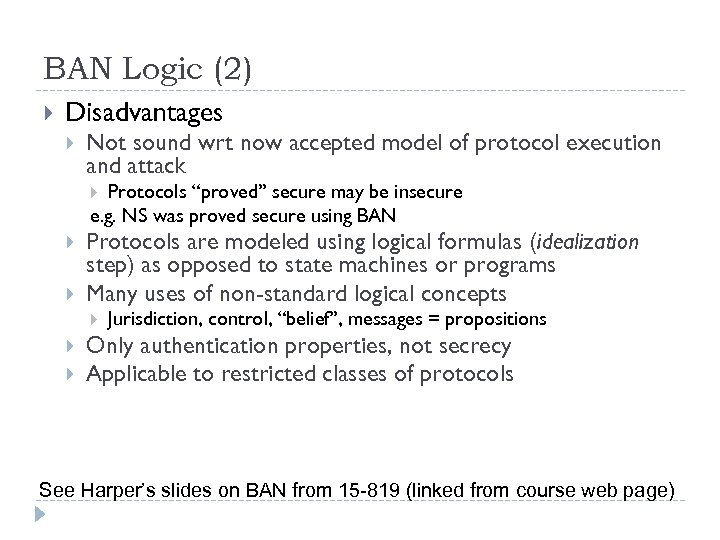BAN Logic (2) Disadvantages Not sound wrt now accepted model of protocol execution and attack Protocols “proved” secure may be insecure e. g. NS was proved secure using BAN Protocols are modeled using logical formulas (idealization step) as opposed to state machines or programs Many uses of non-standard logical concepts Jurisdiction, control, “belief”, messages = propositions Only authentication properties, not secrecy Applicable to restricted classes of protocols See Harper’s slides on BAN from 15 -819 (linked from course web page)